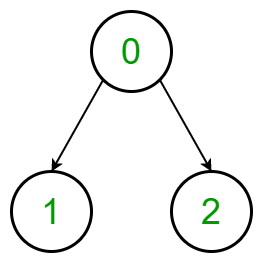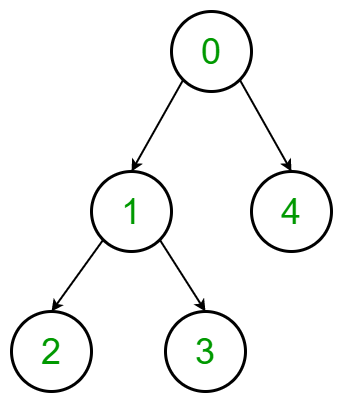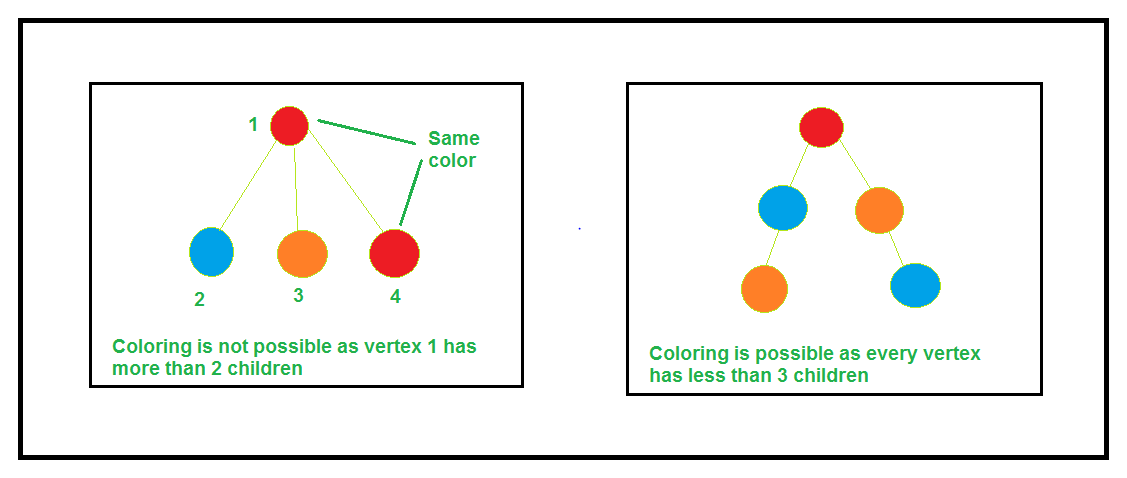Minimum cost to colour a Tree with no 3 adjacent vertices having same colour

• Difficulty Level : Hard
• Last Updated : 21 Jun, 2021

Given a tree with N nodes value from 0 to (N – 1) and a 2D array arr[][] of size dimensions 3xN, where arr[i][j] denotes the cost of coloring jth nodes with color value i. The task is to find the minimum cost of coloring the node of the given tree such that every path of length 3 is colored with distinct colors. If there is any possible way of colouring the nodes of the tree then print the cost else print “Not Possible”.

Examples:

Input: arr[][] = {{3, 2, 3}, {4, 3, 2}, {3, 1, 3}},
Tree:Output:
Explanation:
Color the vertex 0 with type 1 color, cost = 3
Color the vertex 1 with type 3 color, cost = 1
Color the vertex 2 with type 2 color, cost = 2
Therefore the minimum cost is 3 + 1 + 2 = 6

Input: arr[][] = {{3, 4, 2, 1, 2}, {4, 2, 1, 5, 4}, {5, 3, 2, 1, 1}},
Tree:Output: NOT POSSIBLE

Approach: The idea is to make an observation. We need to observe that the answer is not possible if there is a vertex that has more than two edges. The answer exists only for a chain structure, i.e.,• Initially, we check if, for any node, there exist more than two children or not.
• If there exist, then the answer is not possible.
• If there doesn’t exist, then there are only 3P2 available permutations. So, simply check for all the six possible permutations and find the minimum cost.

Below is the implementation of the above approach:

C++

 // C++ program to find the// minimum possible cost// to colour a given tree #include using namespace std; // Class to define a treeclass tree {    vector > g;    vector chain;    int minimum; public:    // Constructor    tree(int n)    {        g = vector >(n);        minimum = 1e6;    }     // Function for pushing edges    void addEdge(int u, int v)    {        g[v].push_back(u);        g[u].push_back(v);    }     // Dfs function to make the chain    // structure of tree in a vector    void dfs(int v, int p = -1)    {        chain.push_back(v);         for (auto i : g[v]) {            if (i == p)                continue;             dfs(i, v);        }    }     // Function that checks all the    // six different type of    // coloring and find the    // minimum of them    void check(int n, int a, int b,               vector > cost)    {        int sum = 0;        vector res(n);         // Assign the color type 1        // to the first element        res = a;         // Assign the color type 2        // to the second element        res = b;         // Add the cost of the color        // of the first element        sum += cost[a][chain];         // Add the cost of the color        // of the second element        sum += cost[b][chain];         for (int i = 2; i < n; i++) {             // Assign the next element in chain            // with different color            res[i] = 3 - res[i - 1] - res[i - 2];             // Add the cost of the element color            sum += cost[res[i]][chain[i]];        }         // Finding the minimum from all cases        if (sum < minimum)            minimum = sum;    }     // Function to find the    // minimum possible cost    // to colour a given tree    void minimumCost(int n,                     vector > cost)    {        for (int i = 0; i < n; i++) {             // Condition to check if            // any vertex consists more than            // 2 edges, then the coloring of            // the vertices is not possible            if (g[i].size() > 2) {                cout << "NOT POSSIBLE"                     << "\n";                return;            }        }         int start;         // Find the starting/ending vertex        for (int i = 0; i < n; i++) {            if (g[i].size() == 1)                start = i;        }         // Call dfs function starting from        // the start vertex        dfs(start);         // Check for all six different        // possible cases        check(n, 0, 1, cost);        check(n, 0, 2, cost);        check(n, 1, 0, cost);        check(n, 1, 2, cost);        check(n, 2, 0, cost);        check(n, 2, 1, cost);         // Printing the minimum cost        cout << minimum << "\n";    }}; // Driver codeint main(){    tree t(5);     t.addEdge(0, 1);    t.addEdge(1, 2);    t.addEdge(2, 3);    t.addEdge(3, 4);     vector > arr        = { { 3, 4, 2, 1, 2 },            { 4, 2, 1, 5, 4 },            { 5, 3, 2, 1, 1 } };     t.minimumCost(5, arr);     return 0;}

Python3

 # Python3 program to find the# minimum possible cost# to colour a given tree # Class to define a treeclass tree:         def __init__(self, n):        self.g = [[] for i in range(n)]        self.minimum = 1000000        self.chain = []      # Function for pushing edges    def addEdge(self, u, v):        self.g[v].append(u);        self.g[u].append(v);             # Dfs function to make the chain    # structure of tree in a vector    def dfs(self, v, p = -1):           self.chain.append(v);              for i in self.g[v]:                  if (i == p):                continue;              self.dfs(i, v);      # Function that checks all the    # six different type of    # coloring and find the    # minimum of them    def check(self, n, a, b, cost):             sum = 0;        res=[0 for i in range(n)]          # Assign the color type 1        # to the first element        res = a;          # Assign the color type 2        # to the second element        res = b;          # Add the cost of the color        # of the first element        sum += cost[a][self.chain];          # Add the cost of the color        # of the second element        sum += cost[b][self.chain];                 for i in range(2, n):              # Assign the next element in chain            # with different color            res[i] = 3 - res[i - 1] - res[i - 2];              # Add the cost of the element color            sum += cost[res[i]][self.chain[i]];                  # Finding the minimum from all cases        if (sum < self.minimum):            self.minimum = sum;      # Function to find the    # minimum possible cost    # to colour a given tree    def minimumCost(self, n, cost):                 for i in range(n):              # Condition to check if            # any vertex consists more than            # 2 edges, then the coloring of            # the vertices is not possible            if (len(self.g[i]) > 2):                print("NOT POSSIBLE")                 return;          start = 0          # Find the starting/ending vertex        for i in range(n):                     if (len(self.g[i]) == 1):                start = i;          # Call dfs function staring from        # the start vertex        self.dfs(start);          # Check for all six different        # possible cases        self.check(n, 0, 1, cost);        self.check(n, 0, 2, cost);        self.check(n, 1, 0, cost);        self.check(n, 1, 2, cost);        self.check(n, 2, 0, cost);        self.check(n, 2, 1, cost);             # Printing the minimum cost        print(self.minimum)     # Driver codeif __name__=='__main__':         t=tree(5);      t.addEdge(0, 1);    t.addEdge(1, 2);    t.addEdge(2, 3);    t.addEdge(3, 4);      arr = [[ 3, 4, 2, 1, 2 ],            [ 4, 2, 1, 5, 4 ],            [ 5, 3, 2, 1, 1 ]];      t.minimumCost(5, arr);  # This code is contributed by rutvik_56

Javascript


Output:
9

Time Complexity: O(N), where N is the number of nodes in the tree.

My Personal Notes arrow_drop_up